2023/7/11

# 什么是Hash

Hash，即散列函数，或者叫哈希函数。它可以`任意长度`的输入通过算法转换成一个`定长`的输出，这个输出就是`散列值`，或者叫`哈希值`。在后文中称为Hash值。

• 从输出无法推导出输入。
• 散列冲突的概率要尽可能小。
• `数据敏感`，对输入的简单修改会导致输出的巨大差异。

## 为什么不能使用基本数据类型

HashMap存储元素首先调用hashCode()方法，计算其Hash值。若相同，则认为是相同的数据，不存储。如果Hash值不同，则再调用其equals()方法进行比较，若返回true则认为是相同对象，不存储。若返回false则存储。

## HashMap是如何计算Hash的？

``````static final int hash(Object key) {
int h;
return (key == null) ? 0 : (h = key.hashCode()) ^ (h >>> 16);
}
``````

# HashMap

## HashMap的使用

### 简单使用

``````import java.util.HashMap;

public class HashMapUse {
public static void main(String[] args) {
HashMap<String, String> map = new HashMap<>(); //创建HashMap
map.put("key", "value"); // 存入值
map.put("erick", "ren");
map.put(null, "yunliyunwai");
map.get("key"); // 取出并打印值 -> value
map.isEmpty(); // 检查map是否为空 -> false
map.containsKey("key"); // 检查map是否存在该键 -> true
map.containsValue("valuee"); // 检查map是否存在该值 -> false
map.values(); // map中所有值 -> [ren, yunliyunwai, value]
map.size(); // map大小 -> 3
map.keySet(); // map中所有键 -> [erick, null, key]
map.entrySet(); // map中所有键与值 -> [erick=ren, null=yunliyunwai, key=value]
HashMap<String, String> map2 = new HashMap<>();
map2.put("nihao", "hello");
map.putAll(map2); // 将新map2追加到map中
System.out.println(map); // -> {erick=ren, null=yunliyunwai, nihao=hello, key=value}
map.remove("erick"); // 删除map中ren键（包括值）
map.remove("key", "valuee"); // 另一种方法(JDK1.8新增)，但是请注意，如果key与value对不上的话，是不会删除成功的。
System.out.println(map); // -> {null=yunliyunwai, nihao=hello, key=value}
Object map3 = map.clone(); // 克隆map数组。注意：此处map3必须是Object类型
System.out.println(map3); // -> {null=yunliyunwai, nihao=hello, key=value}
map.clear(); // 清空map
System.out.println(map); // -> {}
}
}
``````

### JDK1.8新增方法

``````public class JDK8HashMap {
public static void main(String[] args) {
HashMap<String, Integer> map = new HashMap<>();
map.put("key", 100);
map.putIfAbsent("key", 200); // 若map中不存在key即插入，若存在则不作处理
map.putIfAbsent("newkey", 300);
System.out.println(map); // -> {newkey=300, key=100}
map.compute("key", (key, value)-> value - value * 10 / 100); // 重新计算并赋值key,注意：这里使用了Lambda表达式，本质上这里应该是一个BiFunction函数式接口
map.compute("abc", (key, value)-> value - value * 10 / 100); // Exception in thread "main" java.lang.NullPointerException: Cannot invoke "java.lang.Integer.intValue()" because "value" is null
System.out.println(map); // -> {newkey=300, key=90}
map.computeIfAbsent("new_key", key -> 200); //当key不存在时，会将该键值对添加到map中
System.out.println(map); // -> {new_key=200, newkey=300, key=90}
map.computeIfAbsent("new_key", key -> 10); // 当key存在时，不作操作
System.out.println(map); // -> {new_key=200, newkey=300, key=90}
map.computeIfPresent("asdasd", (key, value) -> value - value * 20 / 100); // 当键存在时才会执行，否则忽略。
System.out.println(map); // -> {new_key=200, newkey=300, key=90}
}
}
``````

## HashMap的特点

• HashMap查询效率最高的数据结构，但这是`以空间为代价换来的`（以空间换时间）。
• HashMap可以将null作为键和值。`请注意：ConcurrentHashMap不可以将null作为键或者值的。`
• HashMap不论任何版本都线程不安全

## HashMap数据结构

### JDK1.7

#### 头插是什么意思？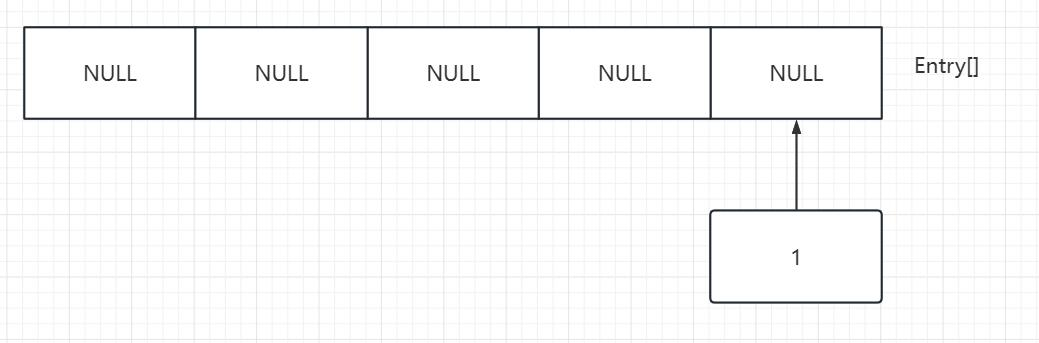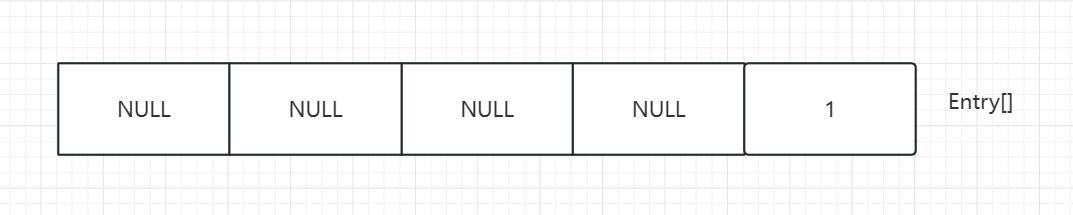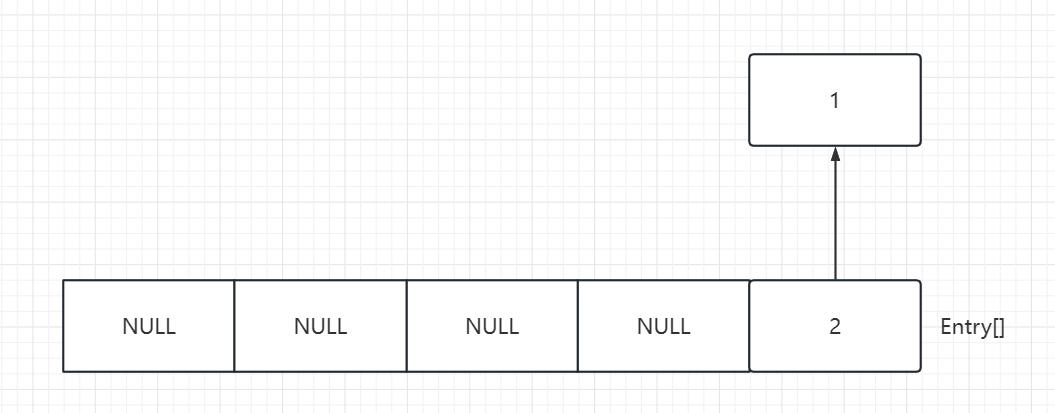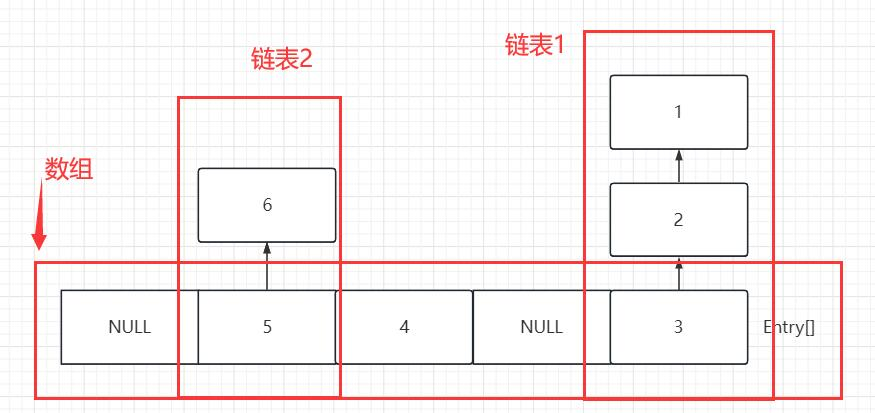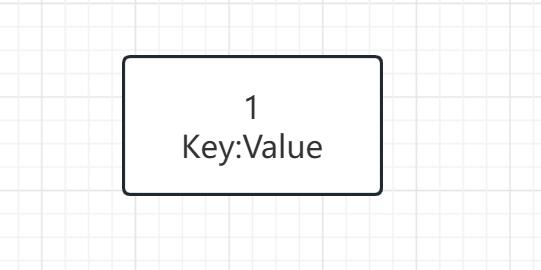### JDK1.8

JDK1.8的HashMap数据结构与1.7基本相同，但在链表长度大于8，`且`Node[]数组（在1.7叫Entry[]）长度大于64时，会将该部分链表转化为红黑树。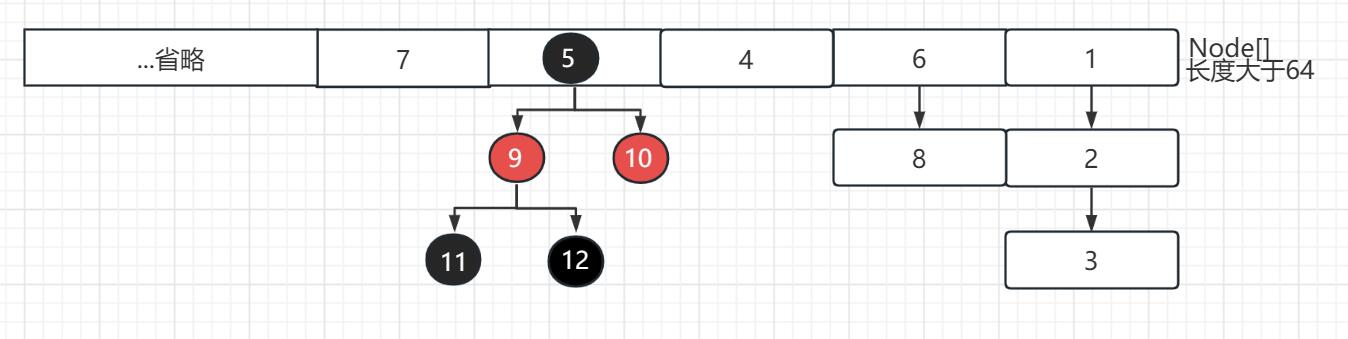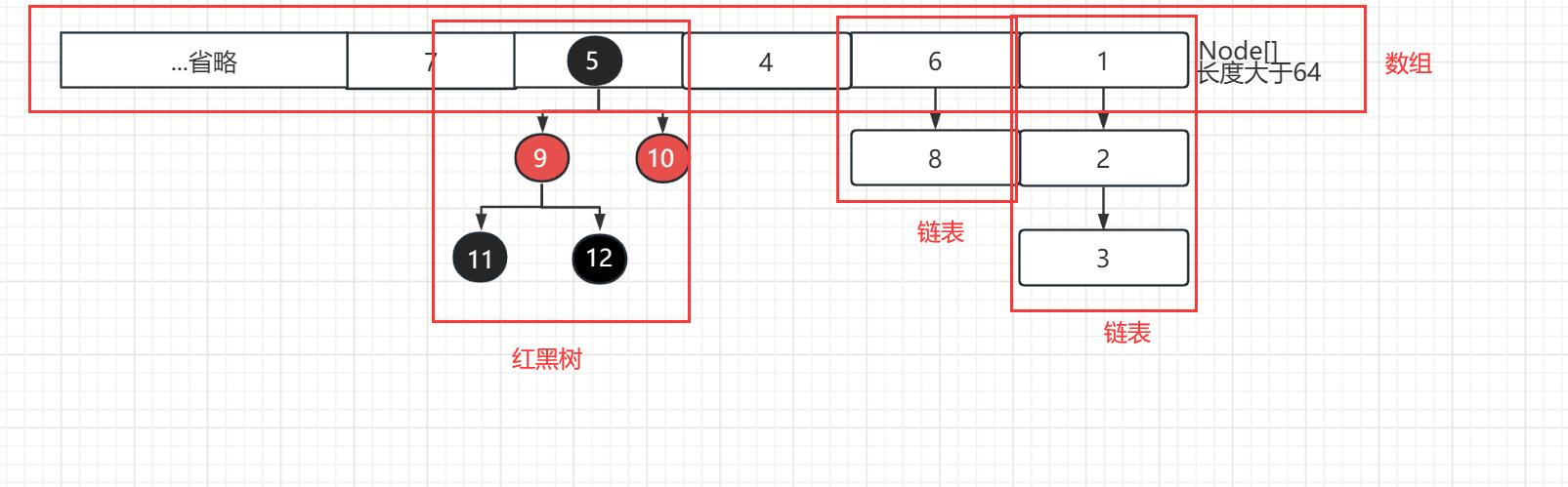### HashMap的扩容机制

#### 扩容机制

HashMap的扩容机制有以下步骤：

##### JDK1.7
• 创建一个新的Entry数组，长度为原来的2倍。(实质上是当前容量左移一位)（newtableSize = tableSize << 1）
• 将原来数组的每个元素重新计算Hash值并放入新的数组中。
##### JDK1.8
###### 如何通过Hash值计算index

``````p = tab[i = (n - 1) & hash]
``````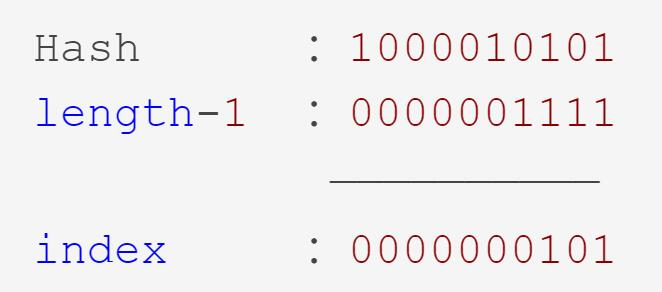###### 高低位算法

JDK1.8中引入了高低位算法，其扩容时引用高低位机制。Node数组中某个位置的链表或者红黑树的结点扩容时，不再以1.7的方式计算索引位置，而是将Node结点的Hash值与旧数组长度进行与运算。`如果计算出来的值为0，表示该结点为低位结点`，将旧数组所有低位结点组合成一个新的Node链表，并赋值到新数组相同位置。`如果计算出来的值不为0，表示该结点为高位结点`，将旧数组所有高位结点组合成一个新的Node链表，赋值给新数组当前位置加上旧数组长度的位置。

Hash值对新容量计算索引值时，后四位不变(因为都是1)，唯一变化的是第五位，即从原来的0变为1。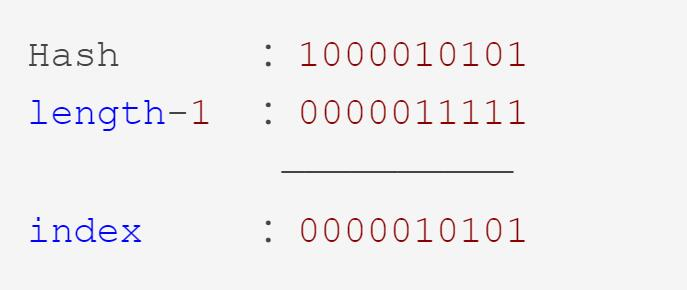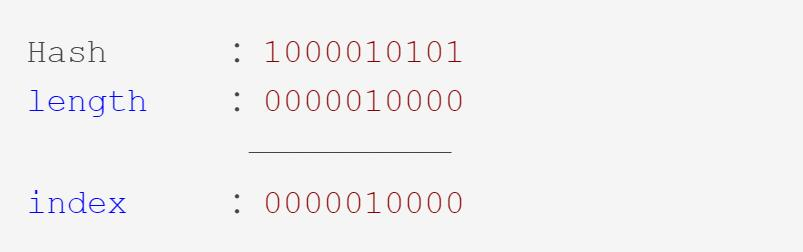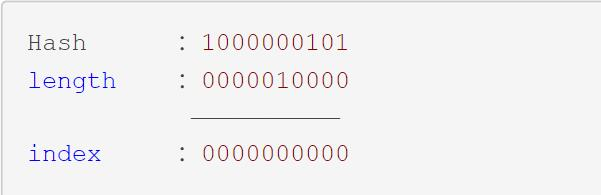###### 扩容过程
• 创建新的Node数组，长度为原来的2倍。
• 依据规律直接按位计算高低位结点，并赋值到新数组对应结点。
###### 为什么要采用高低位算法？

HashMap采用高低位算法主要是为了`减少哈希冲突`，提高哈希表的性能。(因为哈希冲突的部分可能会被重新匀到新的索引位置，而1.7冲突的部分全保留)

HashMap数组的长度是2的幂次方，因此它的长度减1的二进制表示是全1的，如1111、111111等，这样做异或运算后可以保证高位和低位的信息都被保留下来。最终的哈希值也比较均匀地分布在数组的不同位置上，减少哈希冲突的发生，提高了HashMap的性能。

``````static final float DEFAULT_LOAD_FACTOR = 0.75f;
public HashMap() {
}
``````

``````public HashMap(int initialCapacity, float loadFactor) {
if (initialCapacity < 0)
throw new IllegalArgumentException("Illegal initial capacity: " +
initialCapacity);
if (initialCapacity > MAXIMUM_CAPACITY)
initialCapacity = MAXIMUM_CAPACITY;
throw new IllegalArgumentException("Illegal load factor: " +
this.threshold = tableSizeFor(initialCapacity);
}
``````

##### 负载因子为什么默认为0.75

JDK文档：

As a general rule, the default load factor (.75) offers a good tradeoff between time and space costs. Higher values decrease the space overhead but increase the lookup cost (reflected in most of the operations of the HashMap class, including get and put).

#### 初始化容量大小

``````public HashMap(int initialCapacity, float loadFactor) {
if (initialCapacity < 0)
throw new IllegalArgumentException("Illegal initial capacity: " +
initialCapacity);
if (initialCapacity > MAXIMUM_CAPACITY)
initialCapacity = MAXIMUM_CAPACITY;
throw new IllegalArgumentException("Illegal load factor: " +
this.threshold = tableSizeFor(initialCapacity);
}
``````

``````static final int DEFAULT_INITIAL_CAPACITY = 1 << 4; // aka 16
``````

``````static final int MAXIMUM_CAPACITY = 1 << 30;
``````

##### 初始化容量大小为什么默认是16

``````newThr = oldThr << 1;
``````

16是2的整数次幂，可以保证在哈希计算时`只需要进行位运算而不需要乘除法`，提高计算效率。

### HashMap存储流程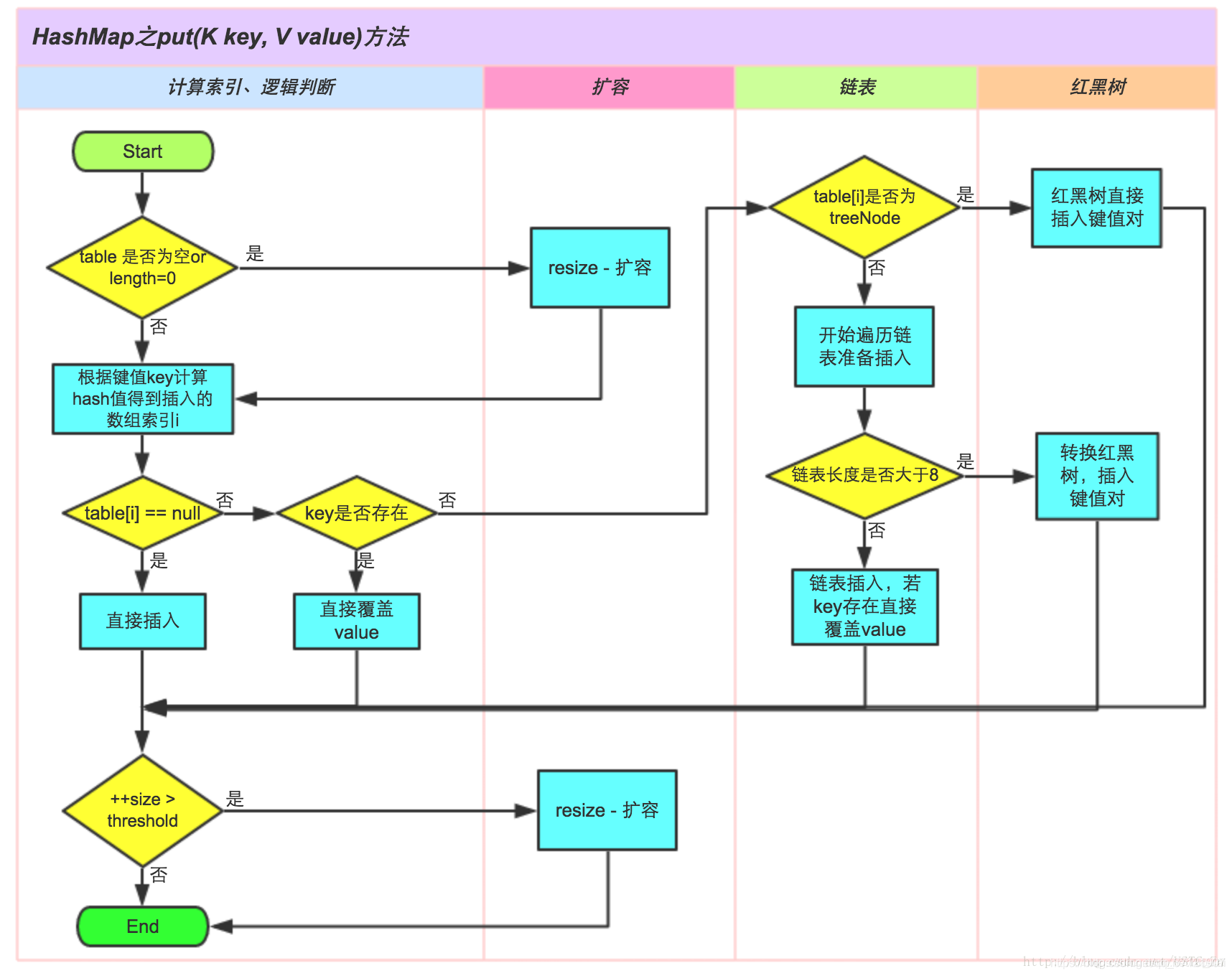## HashMap问题

### 为什么还要使用链表？

`为了避免红黑树的额外空间开销和时间开销`。红黑树需要左旋，右旋，变色这些操作来保持平衡，在链表长度较小时，红黑树的额外空间开销和时间开销可能会超过链表的查询时间复杂度，因此在链表较短时使用链表可以减少不必要的开销。

### 为什么HashMap是无序的？

HashMap实现基于哈希表，哈希表的特点是无序，而链表/红黑树的顺序是根据哈希值和插入顺序决定，所以HashMap没有顺序。

### HashMap的底层数组长度为何总是2的n次幂？

• 使数据分布均匀，减少碰撞
• 当length为2的N次方的时候，索引计算：`h & (length-1) = h % length`，直接使用位运算效率更高。

### null是如何处理的？

HashMap对于键为null的情况，`会将null的hash值设置为0`，所以hash值为0的情况下，`会被映射到数组的第0个索引位置`

``````if (key == null)
return putForNullKey(value);
``````

putForNullkey()方法：

``````private V putForNullKey(V value) {
for (Entry<K,V> e = table; e != null; e = e.next) {
if (e.key == null) {
V oldValue = e.value;
e.value = value;
e.recordAccess(this);
return oldValue;
}
}
modCount++;
return null;
}
``````

## HashMap的线程安全问题

### JDK1.7

#### 1.7的头插法死循环(环状链)问题

JDK1.7采用头插法，在并发执行扩容环境的情况下会造成`环状链(死循环)``数据丢失`的问题。

``````void transfer(Entry[] newTable, boolean rehash) {
int newCapacity = newTable.length;
for (Entry<K,V> e : table) {
while(null != e) {
Entry<K,V> next = e.next;
if (rehash) {
e.hash = null == e.key ? 0 : hash(e.key);
}
int i = indexFor(e.hash, newCapacity);
e.next = newTable[i];
newTable[i] = e;
e = next;
}
}
}
``````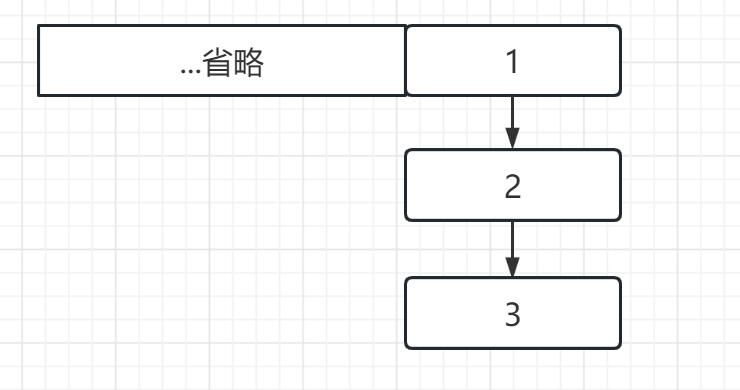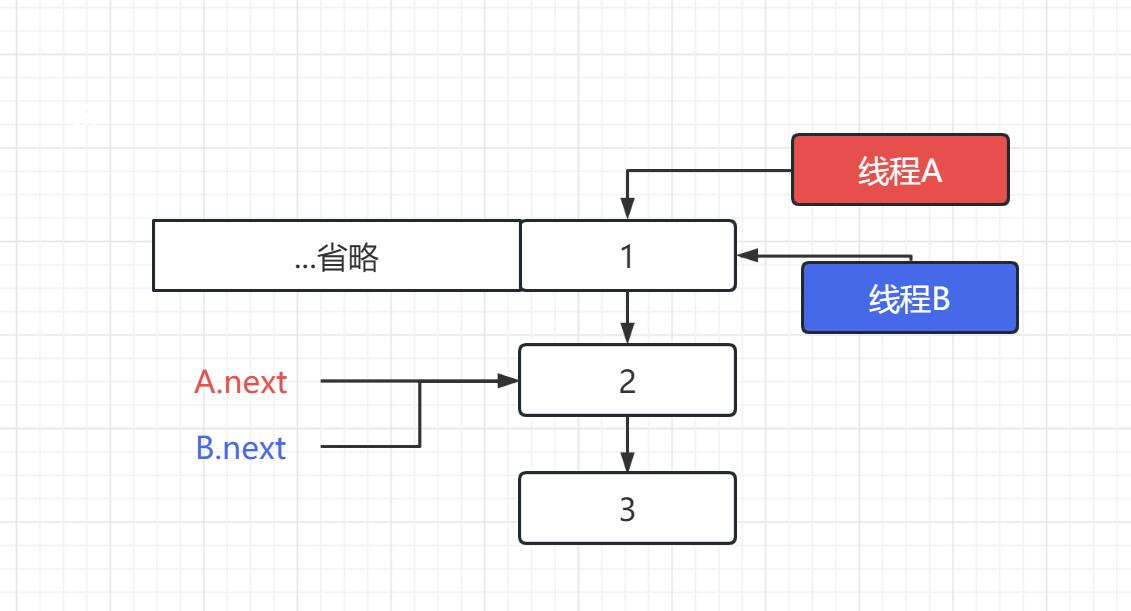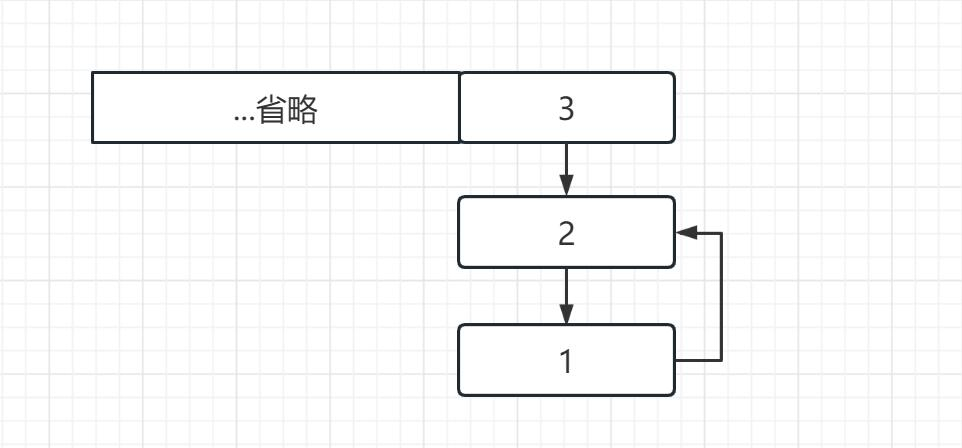### JDK1.8

JDK1.8直接在resize函数中完成了数据迁移，并且使用尾插法以保证链表顺序的一致性，完全修复了死循环问题。

#### 插入数据时数据丢失(覆盖插入)问题

``````final V putVal(int hash, K key, V value, boolean onlyIfAbsent,
boolean evict) {
Node<K,V>[] tab; Node<K,V> p; int n, i;
if ((tab = table) == null || (n = tab.length) == 0)
n = (tab = resize()).length;
if ((p = tab[i = (n - 1) & hash]) == null) //判断哈希碰撞，如果没有碰撞就插入
tab[i] = newNode(hash, key, value, null);
else {
Node<K,V> e; K k;
if (p.hash == hash &&
((k = p.key) == key || (key != null && key.equals(k))))
e = p;
else if (p instanceof TreeNode)
e = ((TreeNode<K,V>)p).putTreeVal(this, tab, hash, key, value);
else {
for (int binCount = 0; ; ++binCount) {
if ((e = p.next) == null) {
p.next = newNode(hash, key, value, null);
if (binCount >= TREEIFY_THRESHOLD - 1) // -1 for 1st
treeifyBin(tab, hash);
break;
}
if (e.hash == hash &&
((k = e.key) == key || (key != null && key.equals(k))))
break;
p = e;
}
}
if (e != null) {
V oldValue = e.value;
if (!onlyIfAbsent || oldValue == null)
e.value = value;
afterNodeAccess(e);
return oldValue;
}
}
++modCount;
if (++size > threshold)
resize();
afterNodeInsertion(evict);
return null;
}
``````

#### 1.8也存在死循环问题

##### 真的存在吗？？
``````public static void main(String[] args) {
HashMap<String, String> map = new HashMap<>();
for (int i = 0; i < 1000000; i+=2) {
map.put(Integer.toString(i), Integer.toBinaryString(i));
if (i > 50000 && i % 10000 == 0){
System.out.println("put");
}
}
}, "A").start();

for (int i = 1; i < 1000000; i+=2) {
map.put(Integer.toString(i), Integer.toBinaryString(i));
if (i > 50000 && i % 10000 == 0){
System.out.println("put");
}
}
}, "B").start();
}
System.out.println(map.size());
}
``````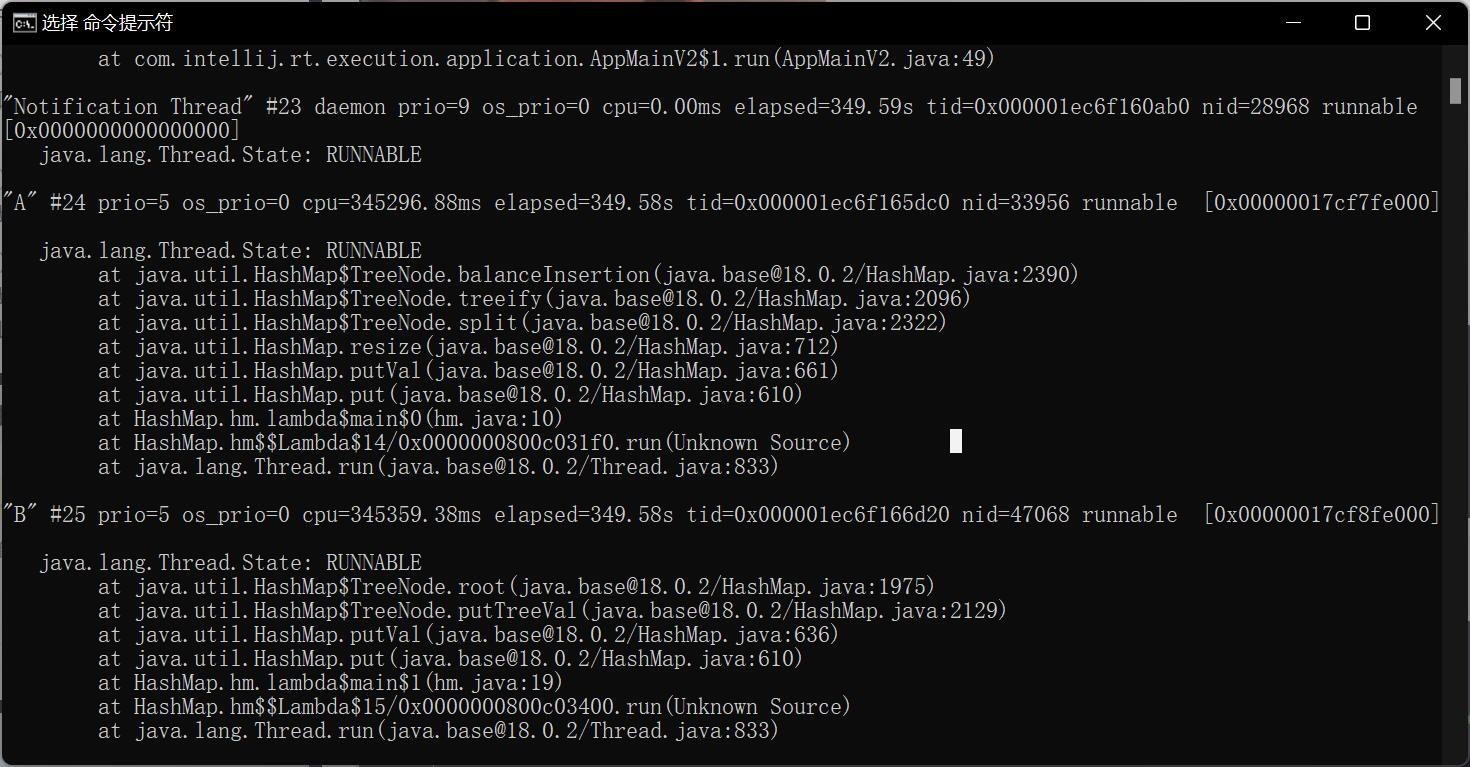`````` while (Thread.activeCount() > 2){
}
``````

## 线程安全问题的解决

### Collections.synchronizedMap转换

``````Map<String, String> map = new HashMap<>();
map = Collections.synchronizedMap(map);
``````

Collections.synchronizedMap()会生成一个同步的Map。

``````public static <K,V> Map<K,V> synchronizedMap(Map<K,V> m) {
return new SynchronizedMap<>(m);
}
``````
``````final Object      mutex;        // Object on which to synchronize

SynchronizedMap(Map<K,V> m) {
this.m = Objects.requireNonNull(m);
mutex = this;
}
``````

``````public V get(Object key) {
synchronized (mutex) {return m.get(key);}
}

public V put(K key, V value) {
synchronized (mutex) {return m.put(key, value);}
}

public V remove(Object key) {
synchronized (mutex) {return m.remove(key);}
}
``````

### ConcurrentHashMap构造

``````ConcurrentHashMap<String, String> map = new ConcurrentHashMap<>();
``````

### 使用HashTable

``````Hashtable<String, String> map = new Hashtable<>();
``````

## 使用

``````HashMap<String, String> hmap = new HashMap<>();
hmap.put("nihao1", "hello");
hmap.put("nihao2", "hello");
hmap.put("nihao3", "hello");
System.out.println(hmap); // -> {nihao3=hello, nihao2=hello, nihao1=hello}
lhmap.put("nihao1", "hello");
lhmap.put("nihao2", "hello");
lhmap.put("nihao3", "hello");
lhmap.get("nihao1");
System.out.println(lhmap); // -> {nihao1=hello, nihao2=hello, nihao3=hello}
lhmap.forEach((key, value) -> {
System.out.println(key + "->" + value);
/*
nihao1->hello
nihao2->hello
nihao3->hello
*/
});
``````

## 插入顺序与访问顺序

`插入顺序即先插入先被遍历`

LinkedHashMap有五个构造方法，只有最后一个构造方法可以传入排序规则，`false为插入顺序，true为访问顺序`

``````public LinkedHashMap(int initialCapacity, float loadFactor) {
accessOrder = false;
}
super(initialCapacity);
accessOrder = false;
}
super();
accessOrder = false;
}
public LinkedHashMap(Map<? extends K, ? extends V> m) {
super();
accessOrder = false;
putMapEntries(m, false);
}
boolean accessOrder//排列规则) {
this.accessOrder = accessOrder;
}
``````

``````LinkedHashMap<String, String> lhmap = new LinkedHashMap<>(16, 0.75f, true);
lhmap.put("nihao1", "hello");
lhmap.put("nihao2", "hello");
lhmap.put("nihao3", "hello");
lhmap.get("nihao1");
lhmap.forEach((key, value) -> {
System.out.println(key + "->" + value);
/*
nihao2->hello
nihao3->hello
nihao1->hello
*/
});
``````

## 实现原理

``````public class LinkedHashMap<K,V>
extends HashMap<K,V>
implements Map<K,V>
``````

`其维护了一个运行于所有条目的双向链表，保证了迭代顺序。`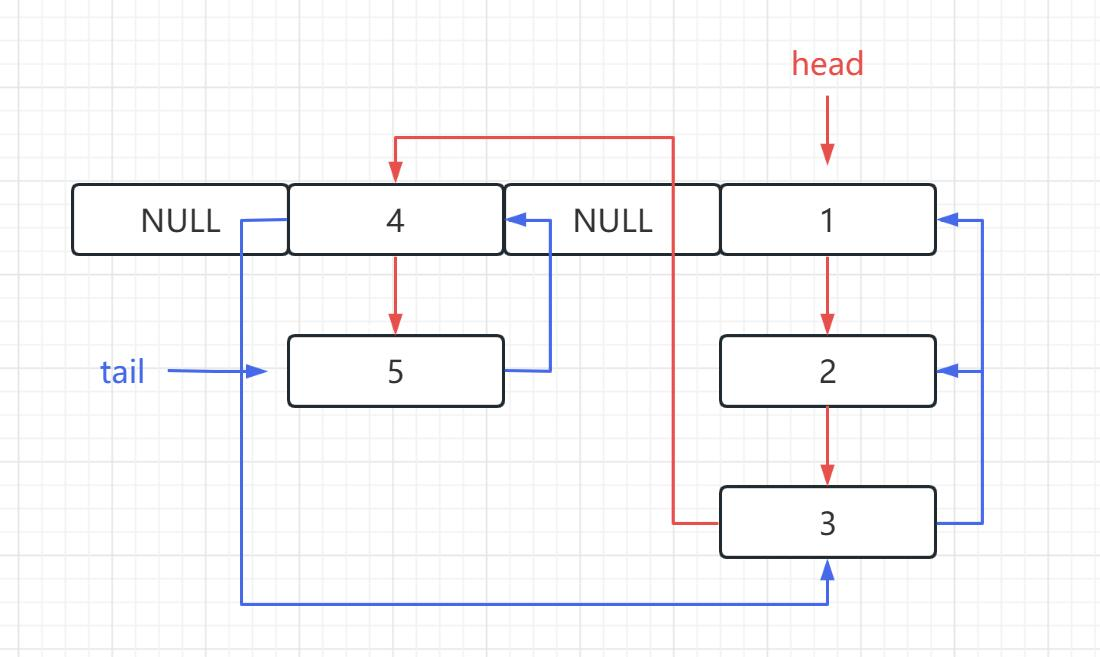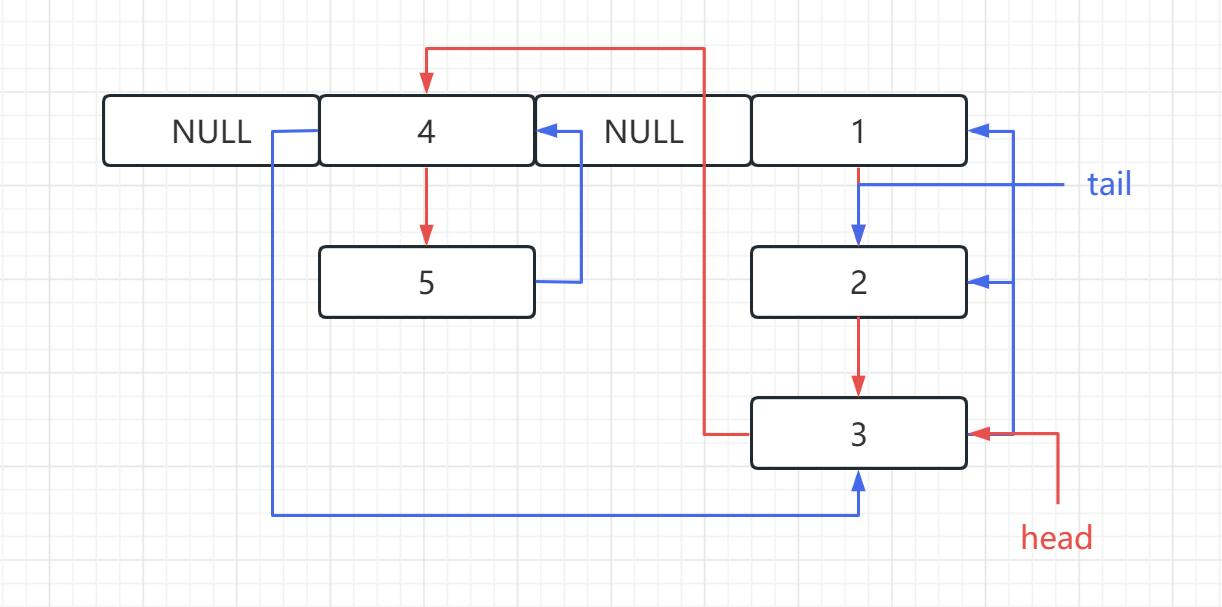## synchronizedMap

``````Map<String, String> lhmap = new LinkedHashMap<>();
lhmap =  Collections.synchronizedMap(lhmap);

lhmap.put("nihao1", "hello");
lhmap.put("nihao2", "hello");
lhmap.put("nihao3", "hello");
lhmap.forEach((key, value) -> {
System.out.println(key + "->" + value);
/*
nihao2->hello
nihao3->hello
nihao1->hello
*/
});
``````

# HashTable

## 与HashMap的区别

• Hashtable在底层仍使用数组+链表的数据结构。

• Hashtable则`不允许`null作为key。

``````if (value == null) {
throw new NullPointerException();
} // put()方法
``````
• Hashtable`初始容量为11`，每次扩容时是容量翻倍并且+1。

``````int newCapacity = (oldCapacity << 1) + 1;
``````
• Hashtable计算hash是直接使用key的hashcode对table数组的长度直接进行取模。(HashMap是对key的hashcode进行了二次hash，以获得更好的散列值，然后对table数组长度取模)

• HashTable产生于JDK 1.1，而HashMap产生于JDK 1.2。

• `Hashtable是线程安全的`

### Hashtable为什么线程安全？

synchronized加锁。

``````public synchronized V put(K key, V value) {
// Make sure the value is not null
if (value == null) {
throw new NullPointerException();
}
...
}

public synchronized V get(Object key) {
Entry<?,?> tab[] = table;
...
return null;
}

public synchronized boolean containsKey(Object key) {
Entry<?,?> tab[] = table;
...
}

......
``````

### 不要在正式代码中使用Hashtable

If a thread-safe implementation is not needed, it is recommended to use HashMap in place of Hashtable. If a thread-safe highly-concurrent implementation is desired, then it is recommended to use java.util.concurrent.ConcurrentHashMap in place of Hashtable.

# HashSet

HashSet是HashMap的衍生类。它储存一个`无序、不重复的集合`，底层由HashMap实现。

## 数据结构

HashSet在内部维护了一个HashMap：

``````private transient HashMap<E,Object> map;

public HashSet() {
map = new HashMap<>();
}
``````

``````public Iterator<E> iterator() {
return map.keySet().iterator();
}

public boolean isEmpty() {
return map.isEmpty();
}

return map.put(e, PRESENT)==null;
}
``````

## HashSet问题

### 底层HashMap中的V为什么有且是一个Object对象常量，为什么不用null？

``````// HashMap插入
final V putVal(int hash, K key, V value, boolean onlyIfAbsent,
boolean evict) {
...
++modCount;
if (++size > threshold)
resize();
afterNodeInsertion(evict);
return null;
}

// HashSet
return map.put(e, PRESENT)==null;
}
``````

``````// HashMap删除
public V remove(Object key) {
Node<K,V> e;
return (e = removeNode(hash(key), key, null, false, true)) == null ?
null : e.value; // 如果removeNode()结果为null，则返回null，否则返回value。
}

final Node<K,V> removeNode(int hash, Object key, Object value,
boolean matchValue, boolean movable) {
...
...
return node; // 正常删除返回node，否则返回null。
}
}
return null;
}

// HashSet
public boolean remove(Object o) {
return map.remove(o)==PRESENT;
}
``````

## HashSet线程不安全

HashSet底层由HashMap实现，所以HashMap的线程不安全同样会表现在HashSet上。

### 解决方法

#### Collections.synchronizedSet()

``````Set<Object> set = Collections.synchronizedSet(new HashSet<>());
``````

#### CopyOnWriteArraySet

``````Set<Object> set = new CopyOnWriteArraySet<>();
``````

CopyOnWriteArraySet内部维护了`CopyOnWriteArrayList`，多数方法由其实现：

``````private final CopyOnWriteArrayList<E> al;

}

public int size() {
return al.size();
}

public boolean isEmpty() {
return al.isEmpty();
}

public boolean contains(Object o) {
return al.contains(o);
}
``````

``````public E set(int index, E element) {
final ReentrantLock lock = this.lock;
lock.lock();
try {
...
} finally {
lock.unlock();
}
}
``````